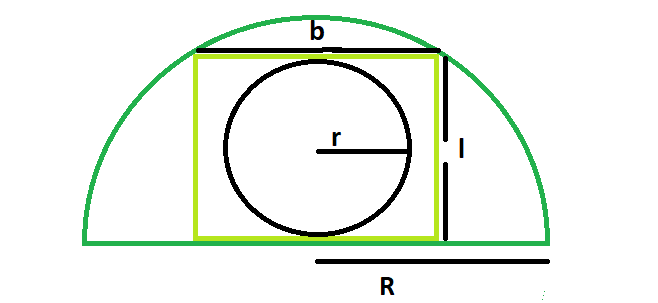# Area of a circle inscribed in a rectangle which is inscribed in a semicircle

Given a semicircle with radius R, which inscribes a rectangle of length L and breadth B, which in turn inscribes a circle of radius r. The task is to find the area of the circle with radius r.

Examples:

```Input : R = 2
Output : 1.57

Input : R = 5
Output : 9.8125
```

## Recommended: Please try your approach on {IDE} first, before moving on to the solution.Approach:

We know the biggest rectangle that can be inscribed within the semicircle has, length, l=√2R/2 &
Also, the biggest circle that can be inscribed within the rectangle has radius, r=b/2=R/2√2(Please refer)
So area of the circle, A=π*r^2=π(R/2√2)^2

## C++

 `// C++ Program to find the area of the circle ` `// inscribed within the rectangle which in turn ` `// is inscribed in a semicircle ` `#include ` `using` `namespace` `std; ` ` `  `// Function to find the area of the circle ` `float` `area(``float` `r) ` `{ ` ` `  `    ``// radius cannot be negative ` `    ``if` `(r < 0) ` `        ``return` `-1; ` ` `  `    ``// area of the circle ` `    ``float` `area = 3.14 * ``pow``(r / (2 * ``sqrt``(2)), 2); ` `    ``return` `area; ` `} ` ` `  `// Driver code ` `int` `main() ` `{ ` `    ``float` `a = 5; ` `    ``cout << area(a) << endl; ` `    ``return` `0; ` `} `

## Java

 `// Java Program to find the area of the circle ` `// inscribed within the rectangle which in turn ` `// is inscribed in a semicircle ` ` `  `import` `java.io.*; ` ` `  `class` `GFG { ` ` `  ` `  `// Function to find the area of the circle ` `static` `float` `area(``float` `r) ` `{ ` ` `  `    ``// radius cannot be negative ` `    ``if` `(r < ``0``) ` `        ``return` `-``1``; ` ` `  `    ``// area of the circle ` `    ``float` `area = (``float``)(``3.14` `* Math.pow(r / (``2` `* Math.sqrt(``2``)), ``2``)); ` `    ``return` `area; ` `} ` ` `  `// Driver code ` ` `  `    ``public` `static` `void` `main (String[] args) { ` `            ``float` `a = ``5``; ` `    ``System.out.println( area(a)); ` `    ``} ` `} ` ` `  ` ``// This code is contributed by ajit `

## Python3

 `# Python 3 Program to find the  ` `# area of the circle inscribed  ` `# within the rectangle which in ` `# turn is inscribed in a semicircle ` `from` `math ``import` `pow``, sqrt ` ` `  `# Function to find the area  ` `# of the circle ` `def` `area(r): ` `     `  `    ``# radius cannot be negative ` `    ``if` `(r < ``0``): ` `        ``return` `-``1` ` `  `    ``# area of the circle ` `    ``area ``=` `3.14` `*` `pow``(r ``/` `(``2` `*` `sqrt(``2``)), ``2``); ` `     `  `    ``return` `area; ` ` `  `# Driver code ` `if` `__name__ ``=``=` `'__main__'``: ` `    ``a ``=` `5` `    ``print``(``"{0:.6}"``.``format``(area(a))) ` ` `  `# This code is contributed By  ` `# Surendra_Gangwar `

## C#

 `// C# Program to find the area of  ` `// the circle inscribed within the  ` `// rectangle which in turn is  ` `// inscribed in a semicircle  ` `using` `System;  ` ` `  `class` `GFG  ` `{  ` ` `  `// Function to find the area ` `// of the circle  ` `static` `float` `area(``float` `r)  ` `{  ` ` `  `    ``// radius cannot be negative  ` `    ``if` `(r < 0)  ` `        ``return` `-1;  ` ` `  `    ``// area of the circle  ` `    ``float` `area = (``float``)(3.14 * Math.Pow(r /  ` `                        ``(2 * Math.Sqrt(2)), 2));  ` `    ``return` `area;  ` `}  ` ` `  `// Driver code  ` `static` `public` `void` `Main (String []args) ` `{  ` `    ``float` `a = 5;  ` `    ``Console.WriteLine(area(a));  ` `}  ` `}  ` ` `  `// This code is contributed ` `// by Arnab Kundu `

## PHP

 `

Output:

```9.8125
```

Attention reader! Don’t stop learning now. Get hold of all the important DSA concepts with the DSA Self Paced Course at a student-friendly price and become industry ready.

My Personal Notes arrow_drop_upCheck out this Author's contributed articles.

If you like GeeksforGeeks and would like to contribute, you can also write an article using contribute.geeksforgeeks.org or mail your article to contribute@geeksforgeeks.org. See your article appearing on the GeeksforGeeks main page and help other Geeks.

Please Improve this article if you find anything incorrect by clicking on the "Improve Article" button below.